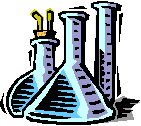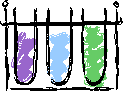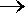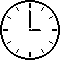Dr. Herrick's CH221 Pressure Quiz 3Stoichiometry Copyright D. Herrick20 Questions from past exams. Practice for speed.  Aim for 2 minutes per problem.
 Constants: Avogadro's number:   6.0221 × 1023
 Atomic weights: H 1.008 O 16.00 C 12.01 F 19.00 N 14.00 Na 23.00
 1 The bond energy for a single hydrogen molecule is 7.17 × 10-19Joule.  The bond energy in kJoule per mole of hydrogen molecules is:
 A) 432 B)  216 C) 387 D) 104 E) 545
 2 Which contains the most oxygen atoms?
 A)  1 mole O B) 1 mole O2 C) 1 mole O3 D) all the same
 3 Which contains the most oxygen atoms?
 A)  1 g O B) 1 g O2 C) 1 g O3 D) all the same4 The weight percent of aluminum in a sample of aluminum oxide is 26.5%.  The weight percent of oxygen is
 A) 49.0 B) 73.5 C) 68.3 D) 79.2 E) 39.6
 5 A compound CxHy contains 92.26 % carbon by weight.  The empirical formula is
 A) C3H8 B) CH C) C2H4 D) CH4 E) C4H6
 6 The molecular formula of a compound (MW = 62.00) that contains 22.58% N and 77.42% O by weight is:
 A)  N2O4 B)  NO C)  NO2 D)  N2O E)  NO3
 7 A hydrate of Fe2O3 contains 59.63% Fe2O3 (MW = 159.68) by weight.  The formula weight of the hydrate is:
 A)  268 B)  304 C)  231 D)  286 E)  250For Questions 8-10 you will first need to balance the equation                       C6H6  +  O2CO2  +  H2O
 8 How many moles of oxygen react to produce 8 moles of water?
 A)  3 B) 20 C) 16 D)  24 E)  9
 9 How many grams of oxygen react with 10 g of C6H6 (MW = 78.11)?
 A)  15.4 B)  183.1 C)  30.7 D) 75.0 E)  61.5
 10 In one experiment the reaction yield was 75%.  How many grams of carbon dioxide (MW = 44.01) were produced from 10 g of C6H6 (MW = 78.11)?
 A) 25.4 B) 16.9 C)  106.5 D) 33.8 E)  50.811 Balance   P4  +  S8P4S10    and determine the moles of P4S10 produced when 0.50 mole of S8 reacts with excess P4.
 A) 0.32 B)  0.50 C)  0.46 D) 0.40 E)  0.54
 12 Identify the limiting reagent when 3 moles A and 7 moles B react by     2 A + 5 B4 C +3 D + E
 A)  A B)  B C)  C D)  D E)  E
 13 Find the moles of CO2 formed when 20 moles of C2H4 and 30 moles of O2 react by    C2H4 + 3 O22 CO2 + 2 H2O
 A)  10 B)  20 C)  30 D)  40 E)  60
 14 Find the grams of CO2 (MW = 44.01) formed when 10.0 g of C2H4  (MW = 28.05) and 10.0 g of oxygen react by    C2H4 + 3 O22 CO2 + 2 H2O
 A) 31.4 B) 20.0 C) 10.5 D) 20.6 E) 9.17
 15 How many moles of HCl are in 2.0 L of a 6.0 M solution of HCl?
 A)  2.0 B)  12 C)  6.0 D)  3.0 E)  0.33
 16 The molarity of a solution containing 165 g of NaCl (MW = 58.45) in 2.34 L of solution is
 A) 1.21 B) 2.50 C) 6.61 D) 0.84 E)  1.00
 17 How many milligrams of NaCl (MW = 58.45) are contained in 25.0 mL of 0.0250 M NaCl solution?
 A) 0.0365 B)  0.625 C) 10.7 D) 36.5 E) 62518 Find the concentration of HCl in a solution prepared by adding water to 200.0 mL of 2.00 M HCl until the volume of the solution is 400.0 mL.
 A) 1.00 M B) 0.100 M C) 0.010 M D) 1.6 M E) 0.160 M
 19 What volume (mL) of 0.167 M HCl (MW = 36.46) contains 128 mg HCl?
 A) 21 D) 781 B) 38.4 E) 621 C) 6.1 F) 14.3
 20 Find the grams of NaCl (MW = 58.45) produced when 250 mL of 0.100M HCl and 50 mL of 0.100 M NaOH react by the neutralization reactionHCl  +  NaOHNaCl  +  H2O
 A)  0.200 B) 1.46 C) 0.412 D)  0.292 E) 1.17